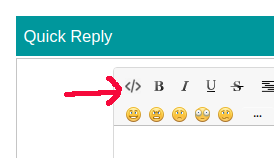# time interval

#define INTERVAL_1 5000
#define INTERVAL_2 7000
#define INTERVAL_3 11000
#define INTERVAL_4 13000

int led1 = 13;
int led2 = 12;
int led3 = 11;
int led4 = 10;

unsigned long time_1 = 0;
unsigned long time_2 = 0;
unsigned long time_3 = 0;
unsigned long time_4 = 0;

void print_time(unsigned long time_millis);

void setup() {
pinMode(led1, OUTPUT);
pinMode(led2, OUTPUT);
pinMode(led3, OUTPUT);
pinMode(led4, OUTPUT);
digitalWrite(led1, LOW);
digitalWrite(led2, LOW);
digitalWrite(led3, LOW);
digitalWrite(led4, LOW);

}

void loop() {
if(millis() > time_1 + INTERVAL_1){
time_1 = millis();
digitalWrite(led1, HIGH);

}

if(millis() > time_2 + INTERVAL_2){
time_2 = millis();
digitalWrite(led2, HIGH);

}

if(millis() > time_3 + INTERVAL_3){
time_3 = millis();
digitalWrite(led3, HIGH);

}

if(millis() > time_4 + INTERVAL_4){
time_4 = millis();
digitalWrite(led4, HIGH);

}
}

how to turn outputs LOW after a 60 seconds of period

need to add a push button at first

thanks

how to turn outputs LOW after a 60 seconds of period

Well, you know when they went HIGH so if you check whether 60 seconds has elapsed since then you can turn them LOW

Have a look at Using millis() for timing. A beginners guide, Several things at the same time and look at the BlinkWithoutDelay example in the IDE.

Don't use millis() like this as it won't work when millis() rolls over

``````if(millis() > time_1 + INTERVAL_1){
``````

Always use subtraction as in

``````if (millis() - time_1 >= INTERVAL_1) {
``````

To get the outputs to go low you use the same technique

``````if (millis() - startTime >= 60000UL) {
// do something
}
``````

Note the use of "UL" to tell the compiler that the number should be treated as unsigned long. All variablesand numbers associated with millis() should be unsigned long.

I don't know, based on your description, whereabouts in the process the value of startTime should be established.

When posting code please use the code button </>``````so your code looks like this
``````

and is easy to copy to a text editor See How to use the Forum

...R

THANKS ,

now clear